Thread: Eric Dollard View Single Postt-rexSenior Member Join Date: Aug 2011 Location: In the bushes Posts: 422
The Law of Electro-Magnetic Induction, Part 5. (2 of 2)

(3) In chapter three, page 16, Steinmetz gives the dynamo formula for the average value of E.M.F. as,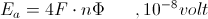Where,

F, frequency in cycles/second.

Steinmetz states here the numeric four results from the lines of force being cut four times in one complete rotation or cycle of alternation. This gives rise to four quarter wave surges of induced E.M.F. Each is in succession, one follows the other. These surges alternate in half wave sets, first plus-minus, then minus plus. For example, consider the 1000 turn reactance coil. At the onset of the cycle the magnetic induction expands outward, this with a north polarity. As it expands it must force its way thru the windings, giving rise to an E.M.F. in a reverse direction. As the cycle progresses the magnetism expands to its maximum extent and then is withdrawn, back inside the coil. This back surge of magnetism must again force its way thru the windings, giving rise to an induced E.M.F. in a forward direction. The first half cycle is now complete, giving a reverse and then a forward induced electro-motive force. At the onset of the next half cycle the magnetic induction expands outward again, this time it is of a south polarity. As it expands it again forces its way thru the windings giving rise to an induced E.M.F. in the forward direction, forward because the magnetism is now south rather than north. As this next half cycle progresses the magnetism expands to its maximum extent and is withdrawn. This back surge of magnetism must again force its way thru the windings to get back inside. This gives rise to an induced E.M.F. in the reverse direction and then the cycle is complete. Hence the existance of four distinct E.M.F. each existing in one quadrant of the A.C. cycle.

1) Expansion, north (+).
2) Contraction, north (-).
3) Expansion, south (-).
4) Contraction, south (+).

Euro-standards require reverse to be positive, its the law, the law of the crank. Reverse means here a back E.M.F. in opposition.

It should be noted that if the windings are shorted with no resistance, it is that no E.M.F. can exist and thus no motion of the magnetic induction is possible. This will halt the rotation of the dynamo.

(4) Steinmetz continues with the development of the dynamo formula. The maximum, or peak, value of E.M.F. is given by the relation,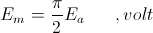This alters the dynamo formula into,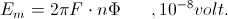Here it is given that,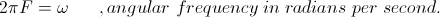Steinmetz fails to incorporate this angular velocity or frequency, into his equations. This would simplify the dynamo equation to,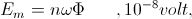or on a Per Turn Basis,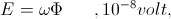Substituting the relation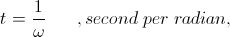The dynamo formula now becomes the basic expression of the law of E.M. Induction, this given as,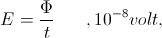This on a per turn, or unit, E.M.F. basis. Hence a volt is given as weber per second. The radian is dimensionless.

Thruout his writings it is that Steinmetz refuses to use the angular frequency,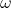, and insists upon the use of the cyclic frequency, F, cycles rather than radians. This unnatural form of expression results in the continued appearance of the factor two pi thruout his equations. This makes each equation that much more complex and gives rise to the situation of interferences with other pi factors causing cancellations and squares.

Steinmetz continues here to give the relation for the effective value of E.M.F.,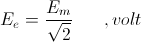This is also known as the root mean square value, (RMS).

The dynamo formula now take the form,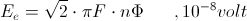This is the Steinmetz Formula for the law of E.M. Induction, a convoluted mess! This is a practitioner's formula for winding dynamo coils, not a starting point for a theoretical study of A.C. phenomena.

BK DE N6KPH

Reference:

Intro to E.M. Theory, Vol I by Oliver Heaviside, note Practitioner.
__________________
SUPPORT ERIC DOLLARD'S WORK AT EPD LABORATORIES, INC.

Purchase Eric Dollard's Books & Videos - 70% of the sale goes to Eric and EPD Laboratories: Eric Dollard Books & Videos
Donate by Paypal: Donate to EPD Laboratories